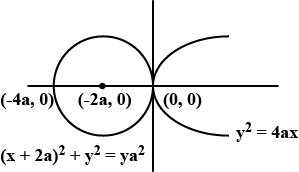QuestionAnswers

# Find the equations to the common tangents of the circle ${x^2} + {y^2} + 4ax = 0$ and parabola ${y^2} = 4ax$

Hint: From the given two equations we first try to find out the center of the circle and its radius. And then we have to find out if the parabola and the circle are touching each other or not. Thus we can find the result by observing the solution.

Here in this problem, we are given two equations, ${x^2} + {y^2} + 4ax = 0$ and ${y^2} = 4ax$. Where one of them is a circle and the other is a parabola. We need to find the equation of common tangents of these two.
So, the equation of the circle is,
${x^2} + {y^2} + 4ax = 0$
Adding $4{a^2}$ to both sides we get,
$\Rightarrow {x^2} + 4ax + 4{a^2} + {y^2} = 4{a^2}$
On applying ${{\text{a}}^{\text{2}}}{\text{ + 2ab + }}{{\text{b}}^{\text{2}}}{\text{ = (a + b}}{{\text{)}}^{\text{2}}}$, we get,
$\Rightarrow {(x + 2a)^2} + {y^2} = 4{a^2}$……(i)
So, we get the circle with center at $( - 2a,0)$and with radius $2a$ units.
And we have the 2nd equation as, ${y^2} = 4ax$ which is passing through the origin.
Now, if we try to plot them altogether, we get,Now, from the figure, it is clearly visible that, the circle and the parabola are touching each other along the y-axis and it is the common tangent of these two given equations.
So, now, the equation of the common tangent is, $x = 0$

Note: In this given problem we focused on finding where the different figures are meeting each other and if they are at all touching each other or not. In these types of problems, if the figures are touching each other, our job becomes easier as the common tangent is always passing through their point of intersection.